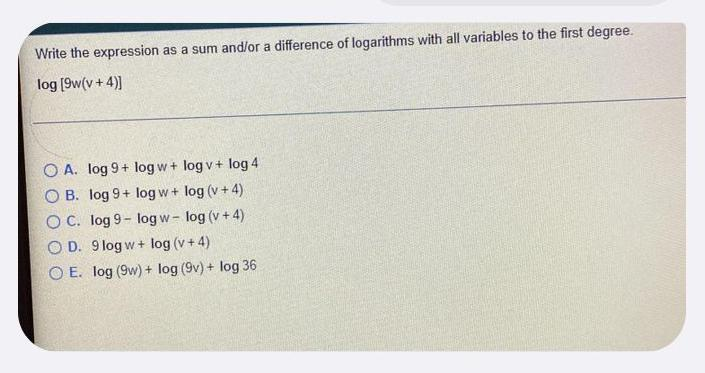Question:

# Write the expression as a sum and/or a difference of logarithms with all variables to the first degree. log [9w(v + 4)] (A) log 9+ log w+ log v + log 4 (B) log 9+ log w+ log (v + 4) (C) log 9- log w-lWrite the expression as a sum and/or a difference of logarithms with all variables to the first degree. log [9w(v + 4)] (A) log 9+ log w+ log v + log 4 (B) log 9+ log w+ log (v + 4) (C) log 9- log w-log (v + 4) (D) 9log w+ log (v + 4) (E) log (9w) + log (9v) + log 36